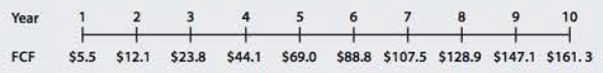Chapter 9, Problem 22SPFundamentals of Financial Manageme...

15th Edition
Eugene F. Brigham + 1 other
ISBN: 9781337395250

Solutions

Chapter
SectionFundamentals of Financial Manageme...

15th Edition
Eugene F. Brigham + 1 other
ISBN: 9781337395250
Textbook Problem

Comprehensive/Spreadsheet ProblemNONCONSTANT GROWTH AND CORPORATE VALUATION Rework problem 9-18, parts a, b, and c, using a spreadsheet model. For part b, calculate the price, dividend yield, and capital gains yield as called for In the problem. After completing parts a through c, answer the following additional question, using the spreadsheet model.d. TTC recently introduced a new line of products that has been wildly successful. On the basis of this success and anticipated future success, the following free cash flows were projected:After the tenth year, TTC’s financial planners anticipate that its free cash flow will grow at a constant rate of 6%. Also, the firm concluded that the new product caused the WACC to fall to 9%. The market value of TTC’s debt is $1,200 million; it uses no preferred stock; and there are 20 million shares of common stock outstanding. Use the corporate valuation model to value the stock.9-18 NONCONSTANT GROWTH STOCK VALUATION Taussig Technologies Corporation (TTC) has been growing at a rate of 20% per year in recent years. This same growth rate is expected to last for another 2 years, then decline to gn =6%. a. If D0 =$1.60 and rs = 10%, what is TIC’s stock worth today? What are its expected dividend, and capital gains yields at this time, that is, during Year 1? b. Now assume that ITC’s period of supernormal growth is to last for 5 years rather than 2 years. Flow would this affect the price, dividend yield, and capital gains yield? Answer in words only. c. What will TTC’s dividend and capital gains yields be once its period of supernormal growth ends? (Hint: These values will be the same regardless of whether you examine the case of 2 or 5 years of supernormal growth; the calculations are very easy.) d. Explain why investors are interested in the changing relationship between dividend and capital gains yields over time.

a.

Summary Introduction

To identify: The stock value, expected dividend and capital gain at year 1.

Introduction:

Corporate Value Model:

The model that evaluates a firm on the basis of its future operations and the results of those operations is called corporate valuation model. It is a useful tool to analyze a firm’s stock for investment purposes.

Explanation

The dividend for the first year is $1.92. (Calculated in working note) The dividend for the second year is$2.30. (Calculated in working note)

The horizon value is $60.95. (Calculated in working note) The interest rate is 10%. (Calculated in working note) Formula to calculate the present value of share, P0=Div1(1+r)1+Div2(1+r)2+...+Divn+Horizonvalue(1+r)n Where, • P0 is the stock value. • Div1 is the dividend for the first year. • Div2 is the dividend for the second year. • Divn is the dividend for the third year. • n is the number of years. • r is the interest rate. Calculation of stock value Table (1) Calculation of expected dividend yield is, The dividend for the first year is$1.92. (Calculated in working note)

The stock value is \$64.99545. (Calculated above)

Formula to calculate the expected dividend yield,

Expecteddividendyield=Div1P0

Where,

• P0 is the stock value.
• Div1 is the dividend for the first year.

b.

Summary Introduction

To calculate: The effect in the stock price, dividend yield and capital gain yield if the TTC’s period of supernormal growth is to last five years rather than two years.

c.

Summary Introduction

To identify: The capital gain yield and dividend yield.

d.

Summary Introduction

To calculate: The value of stock by using corporate valuation model.

Still sussing out bartleby?

Check out a sample textbook solution.

See a sample solution

The Solution to Your Study Problems

Bartleby provides explanations to thousands of textbook problems written by our experts, many with advanced degrees!

Get Started

Find more solutions based on key concepts